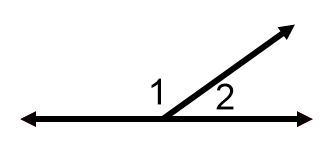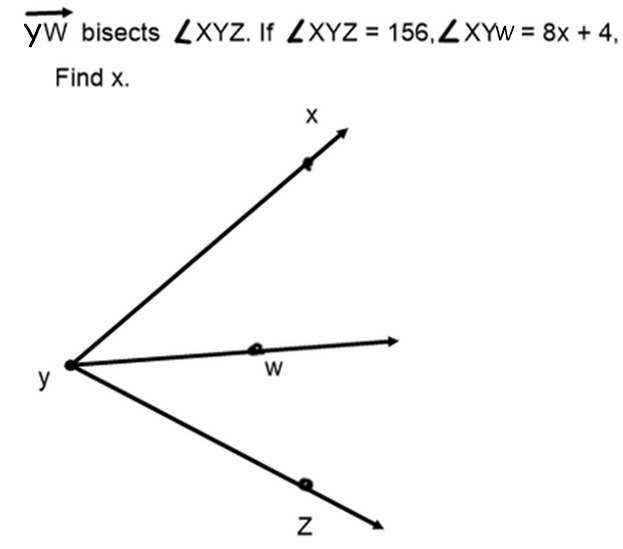Ch1 target 3 Note Application
J is between K and H. Draw, solve, and find x. *
1 point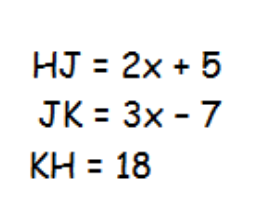C is between Q and R. draw, solve, and find x. *
1 point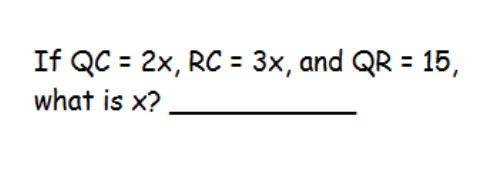Last Name *
Find the angle *
1 point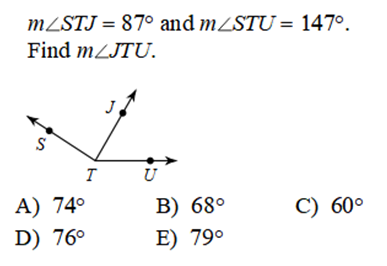Q is the midpoint of segment PR. Find x. *
1 point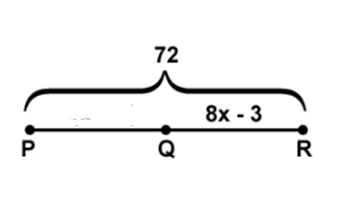Period *
Find x. *
1 point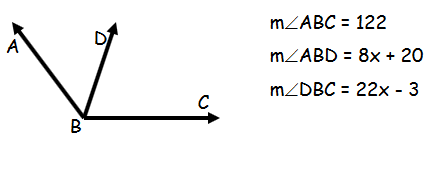Angles A and B together create a 90° angle and ∠A=4x−10 and ∠B=2x−20. Find the measure of angle A. *
1 point
Y is the midpoint of segment xz. FIND THE LENGTH OF XZ!!!! *
1 point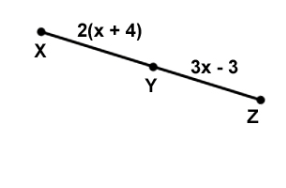Identify the relationship between these two angles *
1 point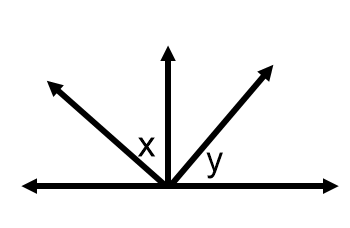Find m<HIJ. *
1 point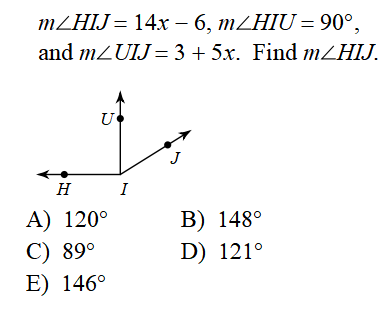Find x. *
1 point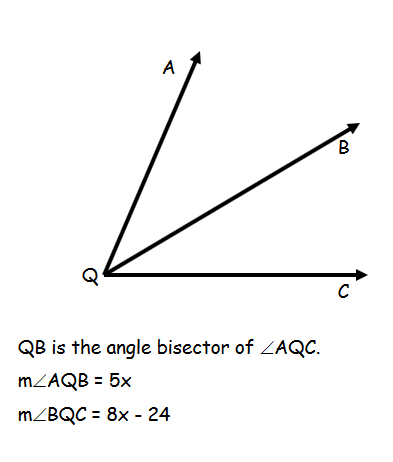Solve for x. *
1 point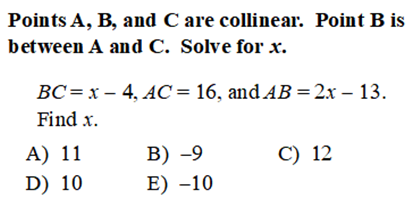Find x. *
1 point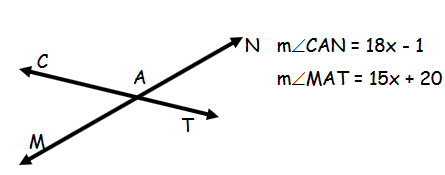Identify the relationship between angles 1 and 2. *
1 point